# simplevis

#### 2020-07-07

library(simplevis)
library(dplyr)

### Making simplevis ggplot graphs

simplevis provides the following types of ggplot graph:

• horizontal bar (e.g. gglot_hbar)
• vertical bar (e.g. gglot_vbar)
• line plot (e.g. gglot_line)
• scatter plot (e.g. gglot_scatter)
• boxplot (e.g. gglot_box)

For each graph type 4 functions are available.

1. A ggplot not coloured or faceted (e.g. gglot_hbar)
plot_data <- ggplot2::diamonds %>%
mutate(cut = stringr::str_to_sentence(cut)) %>%
group_by(cut) %>%
summarise(average_price = mean(price)) %>%
ungroup() %>%
mutate(average_price_thousands = round(average_price / 1000, 1)) %>%
mutate(cut = factor(cut, levels = c("Fair", "Good", "Very good", "Premium", "Ideal")))

plot <- ggplot_hbar(data = plot_data,
x_var = average_price_thousands,
y_var = cut,
title = "Average diamond price by cut",
x_title = "Average price ($US thousands)", y_title = "Cut") plot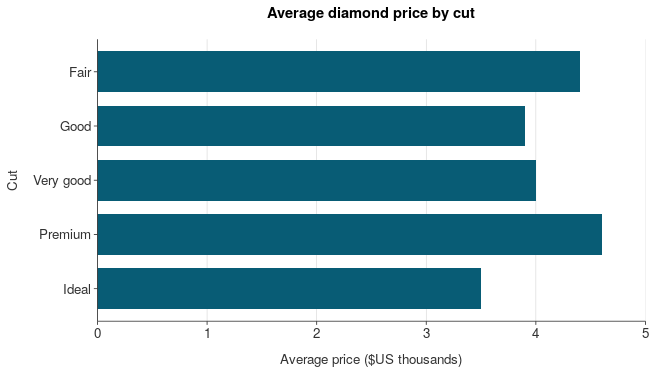1. A ggplot coloured, but not faceted (e.g. gglot_hbar_col) plot_data <- ggplot2::diamonds %>% mutate(cut = stringr::str_to_sentence(cut)) %>% group_by(cut, clarity) %>% summarise(average_price = mean(price)) %>% mutate(average_price_thousands = round(average_price / 1000, 1)) %>% ungroup() plot <- ggplot_hbar_col(data = plot_data, x_var = average_price_thousands, y_var = cut, col_var = clarity, legend_ncol = 4, title = "Average diamond price by cut and clarity", x_title = "Average price ($US thousands)",
y_title = "Cut")

plot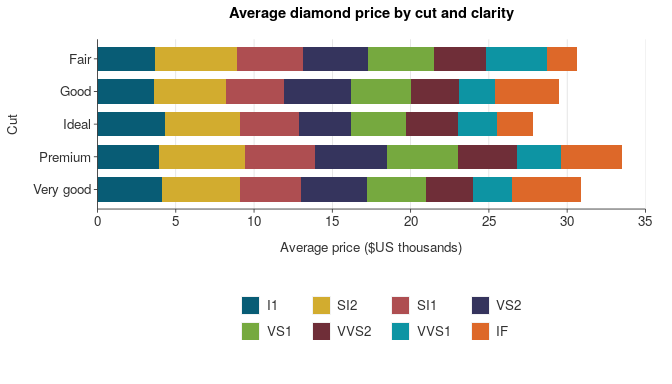1. A ggplot facetted, but not coloured (e.g. gglot_hbar_facet)
plot_data <- ggplot2::diamonds %>%
mutate(cut = stringr::str_to_sentence(cut)) %>%
group_by(cut, clarity) %>%
summarise(average_price = mean(price)) %>%
mutate(average_price_thousands = round(average_price / 1000, 1)) %>%
ungroup()

plot <- ggplot_hbar_facet(data = plot_data,
x_var = average_price_thousands,
y_var = cut,
facet_var = clarity,
title = "Average diamond price by cut and clarity",
x_title = "Average price ($US thousands)", y_title = "Cut") plot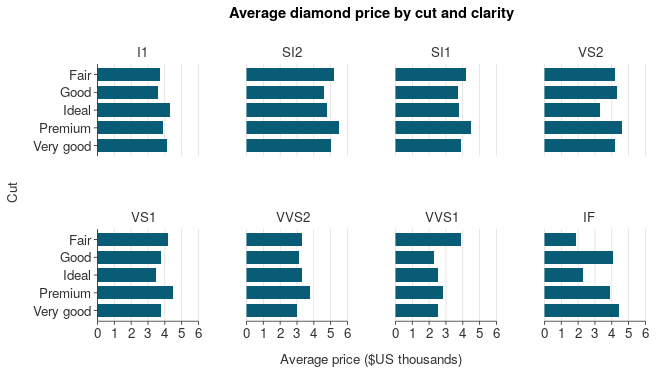1. A ggplot coloured and facetted (e.g. gglot_hbar_col_facet) plot_data <- ggplot2::diamonds %>% mutate(cut = stringr::str_to_sentence(cut)) %>% group_by(cut, clarity, color) %>% summarise(average_price = mean(price)) %>% mutate(average_price_thousands = round(average_price / 1000, 1)) %>% ungroup() plot <- ggplot_hbar_col_facet(data = plot_data, x_var = average_price_thousands, y_var = color, col_var = clarity, facet_var = cut, legend_ncol = 4, title = "Average diamond price by colour, clarity and cut", x_title = "Average price ($US thousands)",
y_title = "Colour")

plot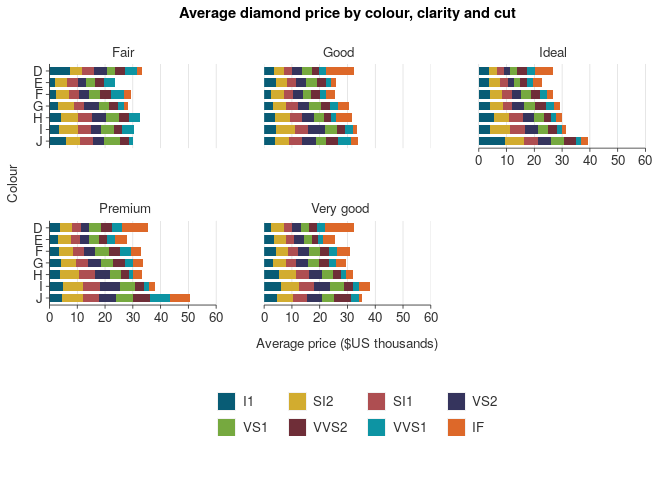These ggplot graphs have been designed that users can convert them easily to html interactive objects by wrapping them in plotly::gglotly with the tooltip = "text" specification. This results in the tooltip converting the applicable variable name to sentence case and replacing underscores with spaces. If users wish to specify a subset of variables, then they need to provide these as a vector instead. Note these variables will turn up in the tooltip as they are specified in the data (e.g. snakecase), unless converted prior in the plot data.

plot_data <- storms %>%
group_by(year) %>%
summarise(average_wind = round(mean(wind), 2)) %>%
ungroup()

plot <- ggplot_vbar(data = plot_data,
x_var = year,
y_var = average_wind,
title = "Average wind speed of Atlantic storms, 1975\u20132015",
x_title = "Year",
y_title = "Average maximum sustained wind speed (knots)")

plotly::ggplotly(plot, tooltip = "text") %>%
plotly_remove_buttons()

plotly::ggplotly(plot, tooltip = c("average_wind")) %>%
plotly_remove_buttons()

### Making simplevis ggplot maps

simplevis provides the following types of ggplot map:

• simple feature (sf) maps
• spatial-temporal array (i.e. stars) maps

Simple feature (sf) maps are maps of points, lines or polygons.

The following functions are available:

• ggplot_sf
• ggplot_sf_col
• ggplot_sf_facet
• ggplot_sf_col_facet

These functions work in the same way as the ggplot graph functions, but with the following key differences:

• Data must be provided in the format of an sf object.
• Data must be of POINT/MULTIPOINT, LINESTRING/MULTILINESTRING, or POLYGON/MULTIPOLYGON geometry types
• Data can have any coordinate reference system (CRS), but it must be defined
• No x_var and y_var variables are required
• There is a coastline specification, which allows for a further sf object as a coastline or administrative boundaries to be added to the map. A New Zealand coastline (nz) and New Zealand coastline with regional boundaries (nz_region) has been provided with the package.
• They do not support conversion to interactive objects through plotly::gglotly.
map_data <- example_sf_nz_river_wq %>%
dplyr::filter(period == "1998-2017", indicator == "Nitrate-nitrogen")

ggplot_sf(data = map_data,
coastline = nz,
size = 0.25,
title = "Monitored river nitrate-nitrogen trend sites, 2008\u201317",
wrap_title = 40)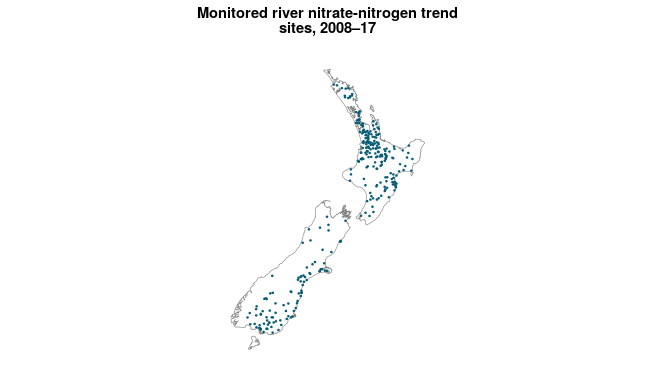map_data <- example_sf_nz_river_wq %>%
filter(period == "1998-2017", indicator == "Nitrate-nitrogen")

pal <- c("#4575B4", "#D3D3D3", "#D73027")

ggplot_sf_col(data = map_data,
col_var = trend_category,
coastline = nz,
size = 0.25,
pal = pal,
title = "Monitored river nitrate-nitrogen trends, 2008\u201317",
wrap_title = 40)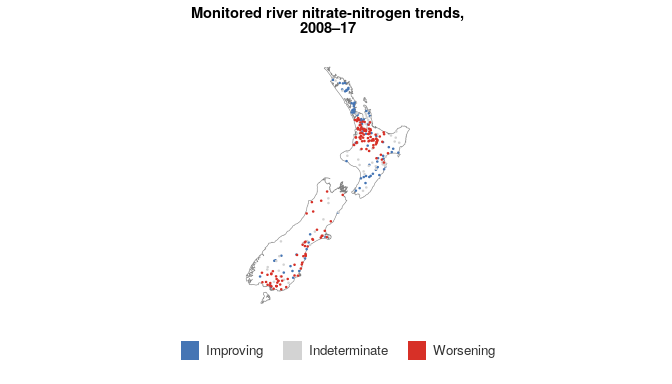map_data <- example_sf_nz_river_wq %>%
filter(period == "1998-2017", indicator == "Nitrate-nitrogen")

ggplot_sf_facet(data = map_data,
facet_var = trend_category,
coastline = nz,
size = 0.25,
title = "Monitored river nitrate-nitrogen trends, 2008\u201317")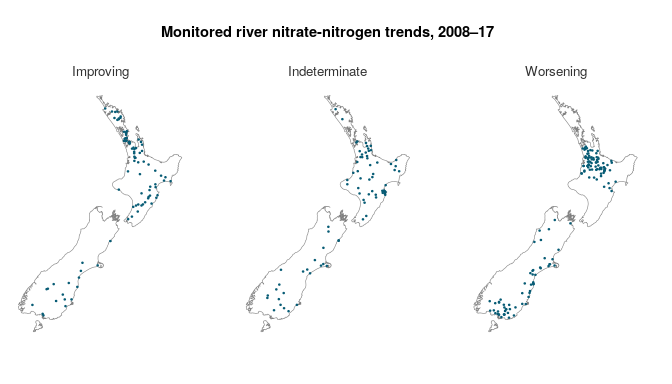map_data <- example_sf_nz_river_wq %>%
filter(period == "1998-2017", indicator %in% c("Nitrate-nitrogen", "Dissolved reactive phosphorus"))

pal <- c("#4575B4", "#D3D3D3", "#D73027")

ggplot_sf_col_facet(data = map_data,
col_var = trend_category,
facet_var = indicator,
coastline = nz,
size = 0.25,
pal = pal,
title = "Monitored river nitrate-nitrogen trends, 2008\u201317")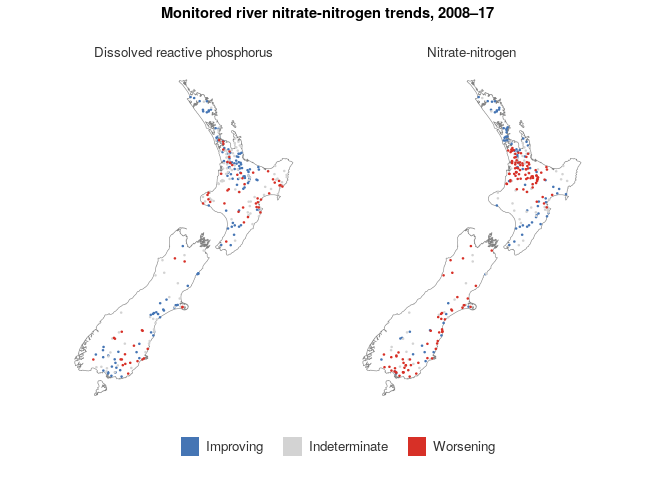simplevis provides ggplot maps made for spatial temporal arrays (stars).

The following functions are available:

• ggplot_sf_col
• ggplot_sf_col_facet

These functions work in the same way as the ggplot sf map functions, but with the following key differences:

• No non-colouring functions have been made, as it is assumed that this functionality is not required
• They do not support conversion to interactive objects through plotly::gglotly.
• Data must be provided in the format of a stars object. For, ggplot_sf_col, the stars object must have 2 dimensions x and y, and only 1 attribute layer. Required input. For, ggplot_sf_col_facet, the stars object must have 2 dimensions, x and y, and multiple named attribute layers with the usual convention of lower case and underscores. Use select, slice, c and splitto get the stars object into the appropriate format.
ggplot_stars_col(data = example_stars_nz_no3n,
coastline = nz,
col_method = "quantile", quantile_cuts = c(0, 0.05, 0.25, 0.5, 0.75, 0.95, 1),
title = "River modelled median nitrate-nitrogen concentrations, 2013\u201317",
wrap_title = 40,
legend_digits = 1)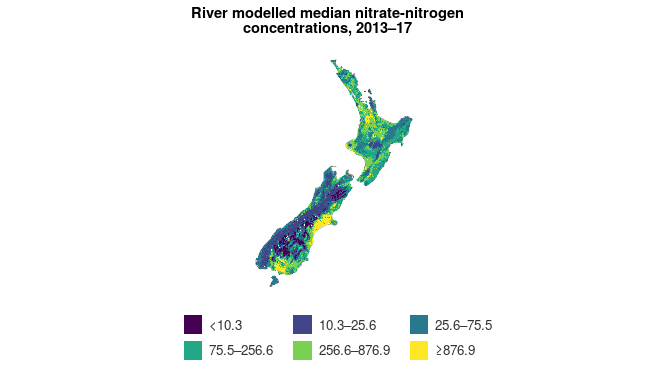map_data1 <- example_stars_nz_no3n %>%
rlang::set_names("NO3N")

map_data2 <- example_stars_nz_drp %>%
rlang::set_names("DRP")

map_data <- c(map_data1, map_data2)

ggplot_stars_col_facet(data = map_data,
coastline = nz,
col_method = "quantile", quantile_cuts = c(0, 0.05, 0.25, 0.5, 0.75, 0.95, 1),
title = "River modelled nutrient concentrations, 2013\u201317")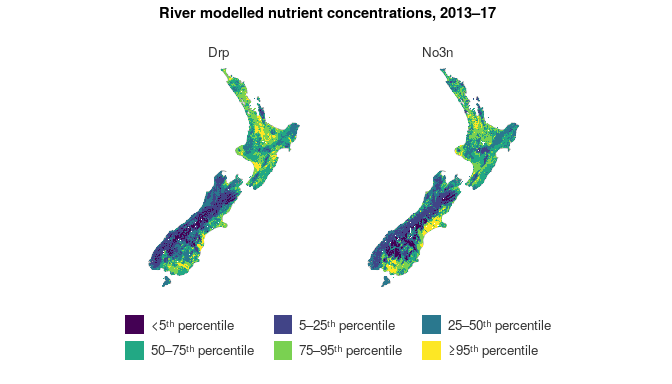### Making simplevis leaflet maps

simplevis provides the following types of leaflet map:

• simple feature (sf) maps
• spatial-temporal array (i.e. stars) maps

These work in the same way as the ggplot map functions, but with no coastline arguments.

Outputs are hidden to keep the size of the vignette manageable.

leaflet_sf(data = example_sf_nz_livestock)
map_data <- example_sf_nz_livestock %>%
mutate(dairydens = round(dairydens, 2))

leaflet_sf_col(data = map_data,
col_var = dairydens,
col_method = "bin",
bin_cuts = c(0, 10, 50, 100, 150, 200, Inf),
legend_digits = 0,
title = "Dairy density in count per km\u00b2, 2017")
leaflet_stars_col(data = example_stars_nz_no3n,
col_method = "quantile", quantile_cuts = c(0, 0.05, 0.25, 0.5, 0.75, 0.95, 1),
title = "River modelled median nitrate-nitrogen concentrations in g/m\u00b3, 2013\u201317")

### Working with quoted variable inputs

simplevis can also work with quoted variable inputs. The user must place each quoted variable within a simplevis function within a !!sym function, as per the example below. This can be helpful, particularly when working in shiny apps.

plot_data <- ggplot2::diamonds %>%
mutate_at(vars("cut"), ~stringr::str_to_sentence(.)) %>%
group_by_at(vars("cut")) %>%
summarise_at(vars("price"), ~mean(.)) %>%
ungroup() %>%
mutate_at(vars("price"), ~round(. / 1000, 2)) %>%
mutate_at(vars("cut"), ~factor(., levels = c("Fair", "Good", "Very good", "Premium", "Ideal")))

x_var <- "price"
y_var <- "cut"

plot <- ggplot_hbar(data = plot_data,
x_var = !!sym(x_var),
y_var = !!sym(y_var),
title = "Average diamond price by cut",
x_title = "Average price ($US thousands)", y_title = "Cut") plot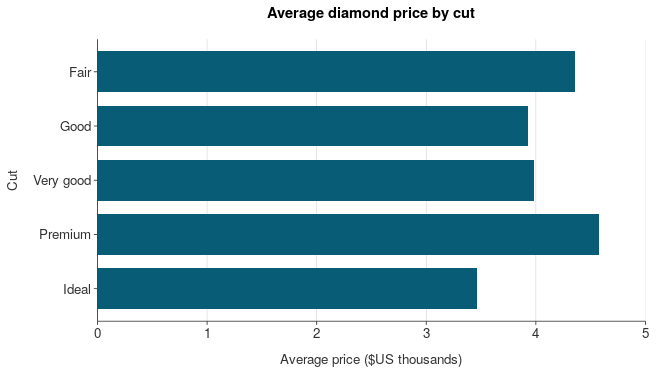### Making shiny apps with simplevis simplevis provides two template shiny apps called template1 and template2. Users can access these functions by using the run_template functions for the applicable app, and then clicking on the download_code button to access a zip file of the code. run_template("template1") # a graph and table run_template("template2") # a leaflet map, as well as graph and table For a simple app, the basic method to create an app is: • run run_template("template1") or run_template("template2") and download the code to use as a template • In make_app_vis.R, draft your visualisations with dummy character inputs • In data subfolder, add your data • In global.R, read your data in, and create any vectors required • In ui.R, add a app title • In ui.R. add radioButtons and other widgets • In server.R, add code within reactive plot_data • In server.R, add code within reactive plot (Note: ensure you have isMobile = input$isMobile within any simplevis ggplot functions, and that simplevis leaflet functions have the argument shiny = TRUE)
• In server.R, check the table is referring to your right dataset
• In server.R, check download code is referring to your dataset. If there are many files, use the download zip code and add a file called download.zip to your data subfolder
• In ui.R, check that the heights of graphs for desktop and mobile are appropriate. (Note: you will need to publish the app and check on your phone before confident that the height for the graph on a mobile device is appropriate)
• In www/About.Rmd, update as necessary
• If using google tag-manager, obtain a tag and replace GTM-XXXXXXX with it in the www/js/tag-manager-js file.

### Mobile compliance - ggplotly for desktop and ggplot for mobile

The template apps have a function with javascript in it that provides a TRUE or FALSE values if the user is on a mobile device. This can value can be referred to in the server code as input$isMobile. This is using the method developed by Gervasio Marchand. The simplevis ggplot functions have a isMobile specification with values of TRUE or FALSE for whether the user is on a mobile device or not. When isMobile equals TRUE, titles, legend elements, and facets are wrapped accordingly for the smaller screen size. Therefore, when simplevis ggplot functions are used within shiny, the app developer must specify isMobile = input$isMobile within the simplevis ggplot function.

The safest option to ensuring that a graph diplays well on a mobile device is to render it as a ggplot object. Subsequently, the current recommended approach used is to render a ggplotly interactive object for desktop users and a ggplot object for mobile users. This is the approach taken by the template apps through the use of conditional panels.

### Iframing

Iframing apps can provide a great experience for users.

Template apps are build to be compatible with one of two approaches to iframing:

• if your website has ability for height responsive iframes, then follow the instructions described in the method developed by Paul Campbell.
• if your website does not support responsive iframes, then templates apps have css that supports a iframe of 750px for desktop users. The app developer can then code the iframe height to be bigger if a mobile user is detected. If this is not possible, a hyperlink to the app url can be provided to mobile users.